# Problems modelling astrometric orbits##Problems modelling astrometric orbits

I've been working on a way to model astrometric orbits, essentially representing 3D orbits on a 2D plane for the purposes of this conversation. I've been having a hard time figuring out the math behind it. I've found what I thought was the proper formulation but it is producing results that are not particularly intuitive.

I will post some screenshots.

A simple, face-on configuration.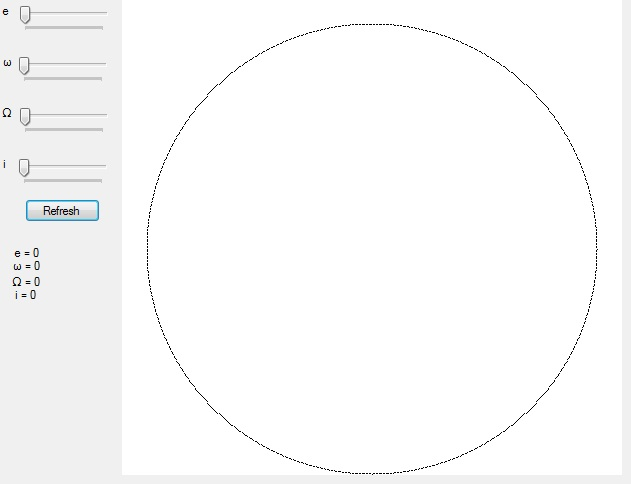As expected, changing LongPeri for an inclined circular orbit does not produce a physical change.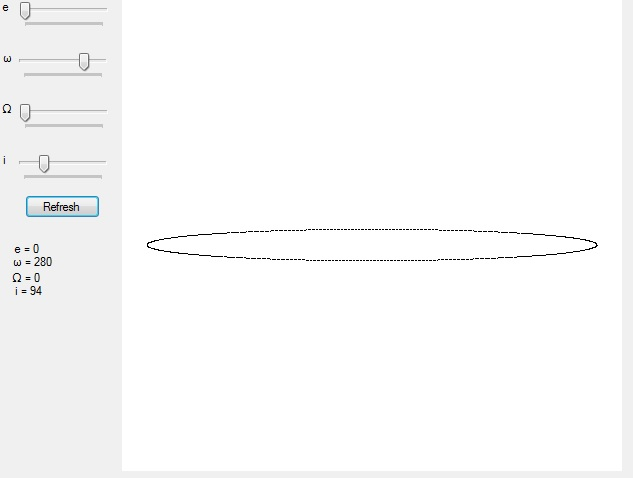But adding eccentricity seems to tilt the orbit.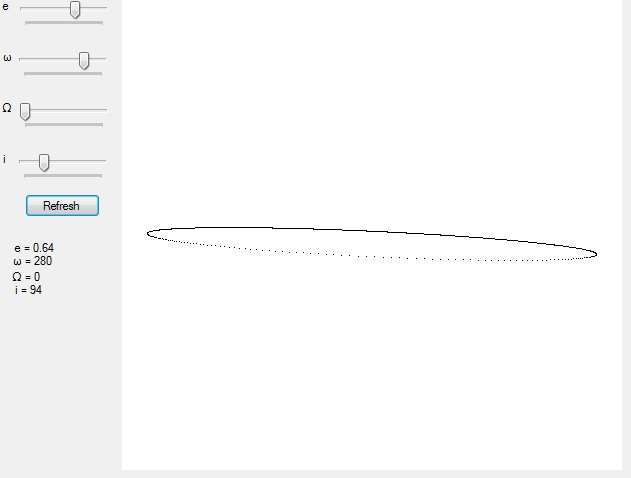Only changing e produces an unexpected result.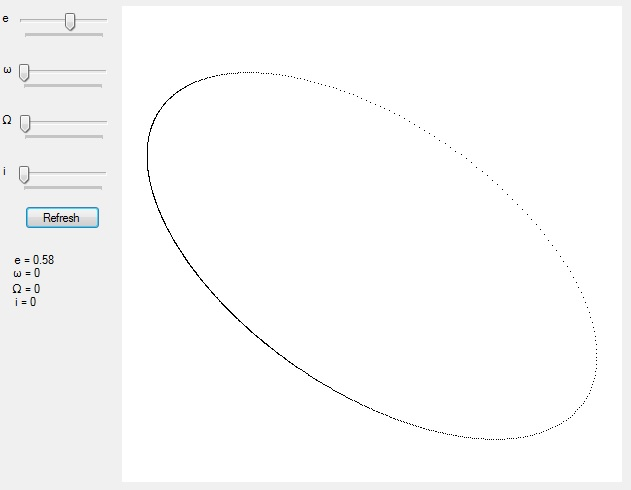Here's my math.
Code:
`//Theile-Innes constants.double A = a * (Math.Cos(omega) * Math.Cos(Node) - Math.Sin(omega) * Math.Sin(Node) * Math.Cos(inc));double B = a * (Math.Cos(omega) * Math.Sin(Node) + Math.Sin(omega) * Math.Cos(Node) * Math.Cos(inc));double F = a * (-1 * Math.Sin(omega) * Math.Cos(Node) - Math.Cos(omega) * Math.Sin(Node) * Math.Cos(inc));double G = a * (-1 * Math.Sin(omega) * Math.Sin(Node) + Math.Cos(omega) * Math.Cos(Node) * Math.Cos(inc));`
Code:
`double x_coord = A * (Math.Cos(E - ecc)) + F * Math.Sqrt(1 - (ecc * ecc)) * Math.Sin(E);double y_coord = B * (Math.Cos(E - ecc)) + G * Math.Sqrt(1 - (ecc * ecc)) * Math.Sin(E);`

I used my same formulation for the eccentric anomaly as used for these diagrams so I do not think it is a problem there.

Also, for eccentric orbits, the x and y coordinates of the orbit are not mapping them to positions that place the barycentre in the image centre.

I'll have to hack at it when I get home from work, but for now I can't figure out what's wrong. Any assistance would be appreciated.

_________________
Caps Lock: Cruise control for 'Cool'!

##Re: Problems modelling astrometric orbits

Follow-up. I've managed to get it working right. The orbit diagrams now match observations for known binary stars, etc.

_________________
Caps Lock: Cruise control for 'Cool'!

##Re: Problems modelling astrometric orbits

Checked out Upsilon Andromedae yet? When I tried to do this a few years back I was unable to match all the data/figures in McArthur et al. (2010) simultaneously.Lazarus
dF star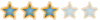Number of posts : 3118
Registration date : 2008-06-12##Re: Problems modelling astrometric orbits